Latest Banking jobs   »   sbi po quant

# Quantitative Aptitude Quiz For SBI PO Prelims 2023 -19th September

Directions (1-5): Please understand the data carefully and answer the following questions.
The pie chart shows distribution of number of students who applied for six different courses, B.Sc, M.Sc, MCA, B.Tech, BCA and BA.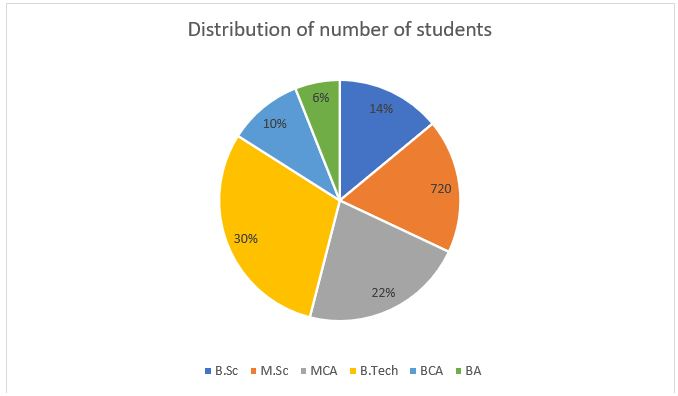Q1. If the ratio of girls to boys who applied for B.Tech course is 2:3, then find the number of boys who applied for B.Tech course?
(a)680
(b)720
(c)640
(d)760
(e)600

Q2. If 25% of the students who applied for MCA did not qualified, then find the number of students who qualified for the MCA course?
(a)720
(b)640
(c)700
(d)680
(e)660

Q3. Find the respective ratio of average number of students who applied for BA & B.Sc to the number of students who applied for M.Sc?
(a)8:5
(b)8:7
(c)5:9
(d)7:8
(e)9:5

Q4. Find the number of students who applied for MCA course is what percent more/less than the number of students who applied for BCA?
(a)130%
(b)115%
(c)125%
(d)120%
(e)110%

Q5. If only 75% of the total students who applied for the different courses are qualified, then find the ratio of number of students who qualified to the number of students who applied for B.Tech & M.Sc together.
(a)25:16
(b)7:9
(c)9:7
(d)16:27
(e)16:9

Directions (6-10): Please understand the data carefully and answer the following questions.
The following line chart shows the distribution of number of students of five different classes, 6, 7, 8, 9 & 10, in three different sections, A, B & C.
Note: Total students in any class = Sum of students in all three sections together.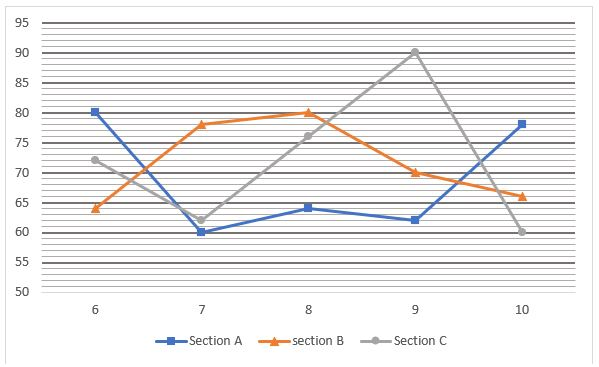Q6. Find the number of students in class 6 & 7 together are approximately what percent more/ less than the number of students in class 8 & 9 together.
(a)8%
(b)7%
(c)4%
(d)5%
(e)6%

Q7. If the respective ratio of boys to girls in section A of class 7 is 2:3, then find the respective ratio of boys in section A of class 7 to the number of students in section B of class 8?
(a)5:3
(b)8:5
(c)3:10
(d)5:8
(e)10:3

Q8. If ‘s’ be the square root of the difference between the total number of students in section A of all five classes and section C of all five classes together, then find the value of 7.5s-10? ?
(a)20
(b)30
(c)10
(d)40
(e)50

Q9. If ‘n’ be the number of students in section C of class 11 and the respective ratio of students in section A, B and C of class 11 is 5:2:4, then find the value of 5n-80, given that total number of students in class 11 is 50% more than the number of students in class 8?
(a)360
(b)420
(c)600
(d)520
(e)560

Q10. Find the respective ratio of total number of students in section B of class 8 & 9 together to the total number of students in class 10?
(a)24:37
(b)25:34
(c)37:24
(d)34:25
(e)24:31

Solutions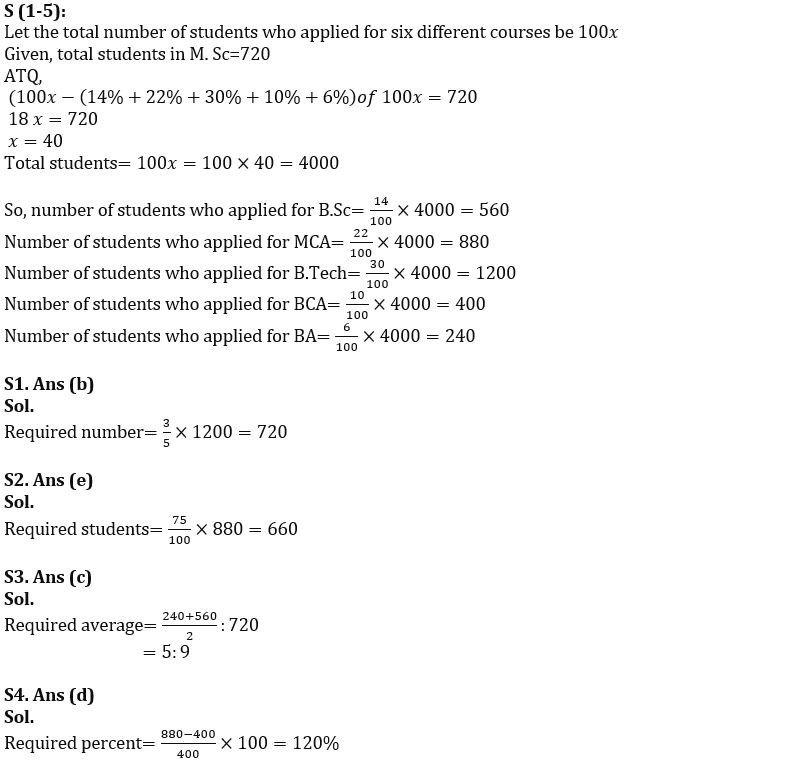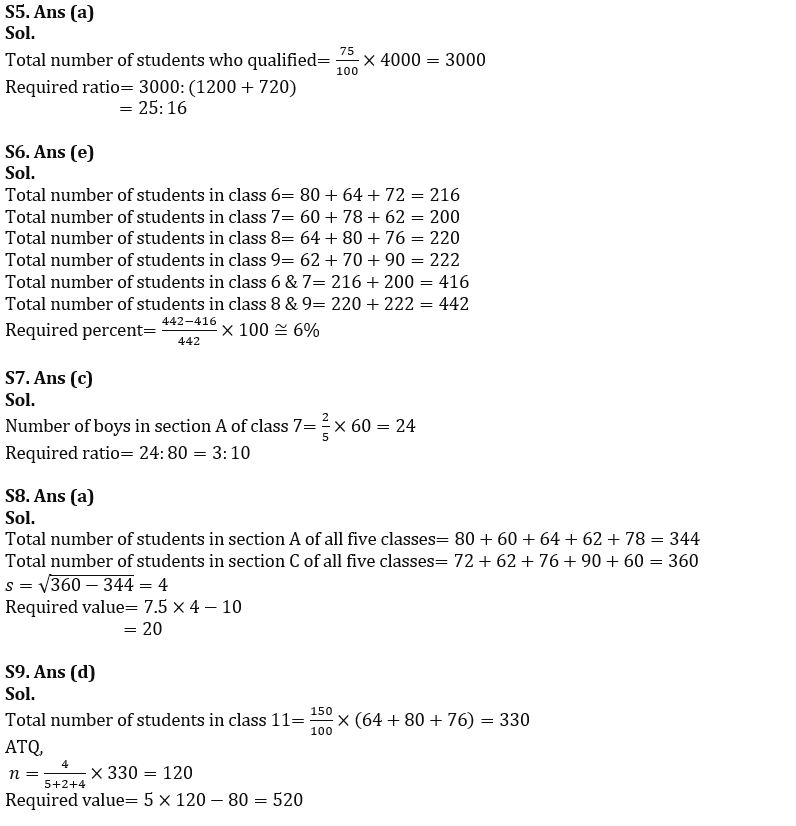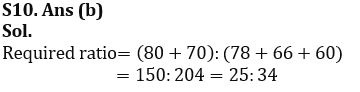. .

## FAQs

### What are the sections asked in the SBI PO Prelims Exam?

SBI PO Prelims exam consists of Reasoning Ability, Quantitative Reasoning and English Language

#### Congratulations!Union Budget 2023-24: Free PDF